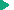SQL Reference
SQL Reference : OpenEdge SQL Language Elements : Data types : Relational operators

Relational operators
Relational operators specify how SQL compares expressions in basic and quantified predicates.

## Syntax

This is the syntax for relational operators:
 = | <> | != | ^= | < | <= | > | >=
The following table lists the relational operators and the resulting predicates for each operator.
 Relational operator Predicate for this relational operator = True if the two expressions are equal. <> | != | ^= True if the two expressions are not equal. The operators != and ^= are equivalent to <>. < True if the first expression is less than the second expression. <= True if the first expression is less than or equal to the second expression. > True if the first expression is greater than the second expression. >= True if the first expression is greater than or equal to the second expression.Basic PredicateQuantified PredicateNumeric arithmetic expressionsDate arithmetic expressions